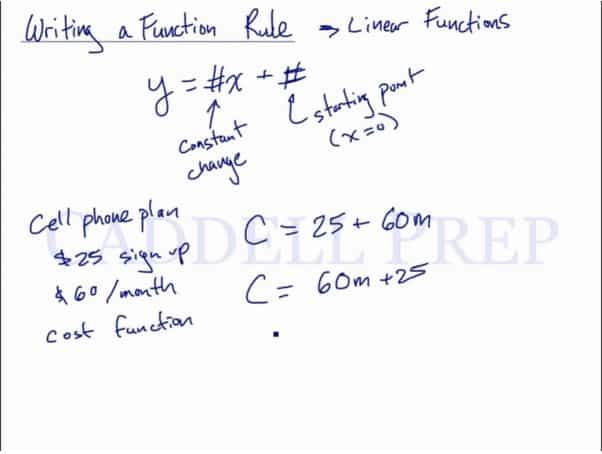This lesson is how to write a function rule. This video shows how functions typically behave, and how to write them down. Functions usually have a variable with a coefficient in front that represents the rate of change and has a constant value that represents the starting point of the function. After you finish this lesson, view all of our Algebra 1 lessons and practice problems.

The setup for this would look like:

Where # represents a constant$y = (\#_1) x + (\#_2)$$(\#_1)$$\rightarrow$ the constant rate of change$(\#_2)$$\rightarrow$ the starting point, x = 0

## Examples of Writing a Function Rule

### Example 1

Jason goes to an amusement park where he pays$\8$ admission and$\2$ per ride. What is the function of the cost?

The cost is$2$ times the number of rides plus$8$. This can be written as a function:$y=2x+8$

### Example 1

Maya has an internet service that currently has a monthly access fee of$\11.95$ and a connection fee of$\0.50$ per hour. Represent her monthly cost as a function of connection time.

The cost has two parts: the one-time fee of$\11.95$ and the per-hour charge of$\0.50$. So the total cost is the charge per hour$\times$ the number of hours$+$ the flat fee$y=0.50x+11.95$

## Video-Lesson Transcript

We’re going to cover how to write a function rule for particularly linear functions.

Linear function has a basic form:$y = (\#_1) x + (\#_2)$

Where the$(\#_1)$ represents the constant rate of change

and$(\#_2)$ is the starting point. The starting point is where$x = 0$.

For example:

We have a cellphone plan that requests$\25$ at sign up and requires$\60$ per month. What is the function of the cost?

It will be cost equals$25$ start-up fee plus$60$ multiplied by the months.

So we’ll have$c = 60m + 25$This relates very closely with the basic form of linear equation which is$y = \#x + \#$.

Let’s look at another example.

A bank account has$\200$ in it. Every week,$\20$ is added to it. Write a function to represent the amount of money (m) in the bank account after (w) weeks.

The money will be equal to starting amount plus the amount you add to it times the number of weeks that passed.$m = 200 + 20w$

After$8$ weeks, the amount of money you have is computed as:$m = 200 + 20(8)$$m = 200 + 160$$m = 360$

Now, let’s see how much money you make after$20$ weeks.

So, we’ll substitute$20$ for$w$.$m = 200 + 20(20)$$m = 200 + 400$$m = 600$

Here, our function can be rewritten as$m = 20w + 200$

which follows the basic linear equation form of$y = \#x + \#$.